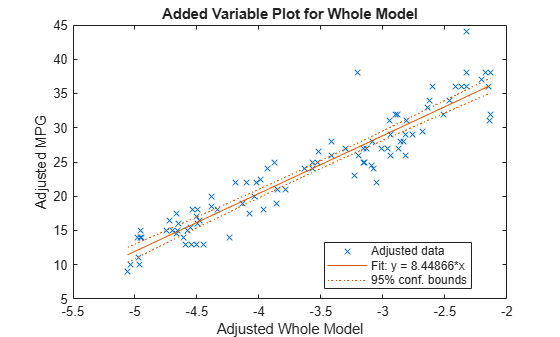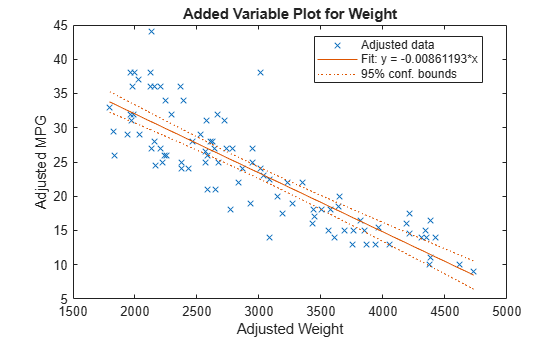Added variable plot of linear regression model

## Syntax

``plotAdded(mdl)``
``plotAdded(mdl,coef)``
``plotAdded(mdl,coef,Name,Value)``
``plotAdded(ax,___)``
``h = plotAdded(___)``

## Description

example

````plotAdded(mdl)` creates an added variable plot for the whole model `mdl` except the constant (intercept) term.```

example

````plotAdded(mdl,coef)` creates an added variable plot for the specified terms `coef`.```
````plotAdded(mdl,coef,Name,Value)` specifies graphical properties of adjusted data points using one or more name-value pair arguments. For example, you can specify the marker symbol and size for the data points.```
````plotAdded(ax,___)` creates the plot in the axes specified by `ax` instead of the current axes, using any of the input argument combinations in the previous syntaxes.```
````h = plotAdded(___)` returns line objects for the plot. Use `h` to modify the properties of a specific line after you create the plot. For a list of properties, see Line Properties.```

## Examples

collapse all

Create a linear regression model of car mileage as a function of weight and model year. Then create an added variable plot to see the significance of the model.

Create a linear regression model of mileage from the `carsmall` data set.

```load carsmall Year = categorical(Model_Year); tbl = table(MPG,Weight,Year); mdl = fitlm(tbl,'MPG ~ Year + Weight^2');```

Create an added variable plot of the model.

`plot(mdl)`The plot illustrates that the model is significant because a horizontal line does not fit between the confidence bounds.

Create the same plot by using the `plotAdded` function.

`plotAdded(mdl)`Create a linear regression model of car mileage as a function of weight and model year. Then create an added variable plot to see the effect of the weight terms (`Weight` and `Weight^2`).

Create the linear regression model using the `carsmall` data set.

```load carsmall Year = categorical(Model_Year); tbl = table(MPG,Weight,Year); mdl = fitlm(tbl,'MPG ~ Year + Weight^2');```

Find the terms in the model corresponding to `Weight` and `Weight^2`.

`mdl.CoefficientNames`
```ans = 1x5 cell {'(Intercept)'} {'Weight'} {'Year_76'} {'Year_82'} {'Weight^2'} ```

The weight terms are 2 and 5.

Create an added variable plot with the weight terms.

```coef = [2 5]; plotAdded(mdl,coef)```The plot illustrates that the weight terms are significant because a horizontal line does not fit between the confidence bounds.

Create a scatter plot of data along with a fitted curve and confidence bounds for a simple linear regression model. A simple linear regression model includes only one predictor variable.

Create a simple linear regression model of mileage from the `carsmall` data set.

```load carsmall tbl = table(MPG,Weight); mdl = fitlm(tbl,'MPG ~ Weight')```
```mdl = Linear regression model: MPG ~ 1 + Weight Estimated Coefficients: Estimate SE tStat pValue __________ _________ _______ __________ (Intercept) 49.238 1.6411 30.002 2.7015e-49 Weight -0.0086119 0.0005348 -16.103 1.6434e-28 Number of observations: 94, Error degrees of freedom: 92 Root Mean Squared Error: 4.13 R-squared: 0.738, Adjusted R-Squared: 0.735 F-statistic vs. constant model: 259, p-value = 1.64e-28 ```

`pValue` of the `Weight` variable is very small, which means that the variable is statistically significant in the model. Visualize this result by creating a scatter plot of the data, along with a fitted curve and its 95% confidence bounds, using the `plot` function.

`plot(mdl)`The plot illustrates that the model is significant because a horizontal line does not fit between the confidence bounds, which is consistent with the `pValue` result.

Create the same plot by using the `plotAdded` function.

`plotAdded(mdl)`When a model includes only one term in addition to the constant term, an adjusted value is equivalent to its original value. Therefore, this added variable plot is the same as the scatter plot created by the `plot` function.

## Input Arguments

collapse all

Linear regression model, specified as a `LinearModel` object created using `fitlm` or `stepwiselm`.

Coefficients in the regression model `mdl`, specified as one of the following:

• Character vector or string scalar of a single coefficient name in `mdl.CoefficientNames` (`CoefficientNames` property of `mdl`).

• Vector of positive integers representing the indexes of coefficients in `mdl.CoefficientNames`. Use a vector to specify multiple coefficients.

Data Types: `char` | `string` | `single` | `double`

Target axes, specified as an `Axes` object.

If you do not specify the axes and the current axes are Cartesian, then `plotAdded` uses the current axes (`gca`). For more information on creating an `Axes` object, see `axes` and `gca`.

### Name-Value Pair Arguments

Specify optional comma-separated pairs of `Name,Value` arguments. `Name` is the argument name and `Value` is the corresponding value. `Name` must appear inside quotes. You can specify several name and value pair arguments in any order as `Name1,Value1,...,NameN,ValueN`.

Example: `'Color','blue','Marker','*'`

### Note

The graphical properties listed here are only a subset. For a complete list, see Line Properties. The specified properties determine the appearance of adjusted data points.

Line color, specified as the comma-separated pair consisting of `'Color'` and an RGB triplet, hexadecimal color code, color name, or short name for one of the color options listed in the following table.

The `'Color'` name-value pair argument also determines marker outline color and marker fill color if `'MarkerEdgeColor'` is `'auto'` (default) and `'MarkerFaceColor'` is `'auto'`.

For a custom color, specify an RGB triplet or a hexadecimal color code.

• An RGB triplet is a three-element row vector whose elements specify the intensities of the red, green, and blue components of the color. The intensities must be in the range `[0,1]`; for example, ```[0.4 0.6 0.7]```.

• A hexadecimal color code is a character vector or a string scalar that starts with a hash symbol (`#`) followed by three or six hexadecimal digits, which can range from `0` to `F`. The values are not case sensitive. Thus, the color codes `'#FF8800'`, `'#ff8800'`, `'#F80'`, and `'#f80'` are equivalent.

Alternatively, you can specify some common colors by name. This table lists the named color options, the equivalent RGB triplets, and hexadecimal color codes.

Color NameShort NameRGB TripletHexadecimal Color CodeAppearance
`'red'``'r'``[1 0 0]``'#FF0000'``'green'``'g'``[0 1 0]``'#00FF00'``'blue'``'b'``[0 0 1]``'#0000FF'``'cyan'` `'c'``[0 1 1]``'#00FFFF'``'magenta'``'m'``[1 0 1]``'#FF00FF'``'yellow'``'y'``[1 1 0]``'#FFFF00'``'black'``'k'``[0 0 0]``'#000000'``'white'``'w'``[1 1 1]``'#FFFFFF'``'none'`Not applicableNot applicableNot applicableNo color

Here are the RGB triplets and hexadecimal color codes for the default colors MATLAB® uses in many types of plots.

`[0 0.4470 0.7410]``'#0072BD'``[0.8500 0.3250 0.0980]``'#D95319'``[0.9290 0.6940 0.1250]``'#EDB120'``[0.4940 0.1840 0.5560]``'#7E2F8E'``[0.4660 0.6740 0.1880]``'#77AC30'``[0.3010 0.7450 0.9330]``'#4DBEEE'``[0.6350 0.0780 0.1840]``'#A2142F'`Example: `'Color','blue'`

Line width, specified as the comma-separated pair consisting of `'LineWidth'` and a positive value in points. If the line has markers, then the line width also affects the marker edges.

Example: `'LineWidth',0.75`

Marker symbol, specified as the comma-separated pair consisting of `'Marker'` and one of the values in this table.

ValueDescription
`'o'`Circle
`'+'`Plus sign
`'*'`Asterisk
`'.'`Point
`'x'`Cross
`'square'` or `'s'`Square
`'diamond'` or `'d'`Diamond
`'^'`Upward-pointing triangle
`'v'`Downward-pointing triangle
`'>'`Right-pointing triangle
`'<'`Left-pointing triangle
`'pentagram'` or `'p'`Five-pointed star (pentagram)
`'hexagram'` or `'h'`Six-pointed star (hexagram)
`'none'`No markers

Example: `'Marker','+'`

Marker outline color, specified as the comma-separated pair consisting of `'MarkerEdgeColor'` and an RGB triplet, hexadecimal color code, color name, or short name for one of the color options listed in the `Color` name-value pair argument.

The default value of `'auto'` uses the same color specified by using `'Color'`.

Example: `'MarkerEdgeColor','blue'`

Marker fill color, specified as the comma-separated pair consisting of `'MarkerFaceColor'` and an RGB triplet, hexadecimal color code, color name, or short name for one of the color options listed in the `Color` name-value pair argument.

The `'auto'` value uses the same color specified by using `'Color'`.

Example: `'MarkerFaceColor','blue'`

Marker size, specified as the comma-separated pair consisting of `'MarkerSize'` and a positive value in points.

Example: `'MarkerSize',2`

## Output Arguments

collapse all

Line objects, returned as a 3-by-1 vector. `h(1)`, `h(2)`, and `h(3)` correspond to the adjusted data points, fitted line, and 95% confidence bounds of the fitted line, respectively. Use dot notation to query and set properties of the line objects. For details, see Line Properties.

You can use name-value pair arguments to specify the appearance of adjusted data points corresponding to the first graphics object `h(1)`.

collapse all

An added variable plot, also known as a partial regression leverage plot, illustrates the incremental effect on the response of specified terms caused by removing the effects of all other terms.

An added variable plot created by `plotAdded` with a single selected term corresponding to a single predictor variable includes these plots:

• Scatter plot of adjusted response values against adjusted predictor variable values

• Fitted line for adjusted response values as a function of adjusted predictor variable values

• 95% confidence bounds of the fitted line

The adjusted values are equal to the average of the variable plus the residuals of the variable fit to all predictors except the selected predictor. For example, consider an added variable plot for the first predictor variable x1. Fit the response variable y and the selected predictor variable x1 to all predictors except x1 as follows:

yi = gy(x2i, x3i, …, xpi) + ryi,

x1i = gx(x2i, x3i, …, xpi) + rxi,

where gy and gx are the fit of y and x1, respectively, against all predictors except the selected predictor (x1). ry and rx are the corresponding residual vectors. The subscript i represents the observation number. The adjusted value is the sum of the average value and the residual for each observation.

`$\begin{array}{c}{\stackrel{˜}{y}}_{i}=\overline{y}+{r}_{yi},\\ {\stackrel{˜}{x}}_{1i}={\overline{x}}_{1}+{r}_{xi},\end{array}$`

where ${\overline{x}}_{1}$ and $\overline{y}$ represent the average of x1 and y, respectively.

`plotAdded` plots a scatter plot of (${\stackrel{˜}{x}}_{1i}$, ${\stackrel{˜}{y}}_{i}$), a fitted line for $\stackrel{˜}{y}$ as a function of ${\stackrel{˜}{x}}_{1}$ (that is, ${\beta }_{1}{\stackrel{˜}{x}}_{1}$), and the 95% confidence bounds of the fitted line. The coefficient β1 is the same as the coefficient estimate of x1 in the full model, which includes all predictors.

ryi represents the part of the response values unexplained by the predictors (except x1), and rxi represents the part of the x1 values unexplained by the other predictors. Therefore, the fitted line represents how the new information introduced by adding x1 can explain the unexplained part of the response values. If the slope of the fitted line is close to zero and the confidence bounds can include a horizontal line, then the plot indicates that the new information from x1 does not explain the unexplained part of the response values well. That is, x1 is not significant in the model fit.

`plotAdded` also supports an extension of the added variable plot so that you can select multiple terms instead of a single term. Therefore, you can also specify a categorical predictor, all terms that involve a specific predictor, or the model as a whole (except a constant (intercept) term). Consider a set of predictors X with a coefficient vector β, where βi is the coefficient estimate of xi in the full model if you specify the ith coefficient for an added variable plot; otherwise, βi is zero. Define a unit direction vector u as u = β/s where s = norm(β). Then, Xβ = (Xu)s. Treat Xu as a single predictor with a coefficient s, and create an added variable plot for Xu in the same way as creating the plot for a single term. The coefficient of the fitted line in the added variable plot corresponds to s.

## Tips

• The data cursor displays the values of the selected plot point in a data tip (small text box located next to the data point). The data tip includes the x-axis and y-axis values for the selected point, along with the observation name or number.